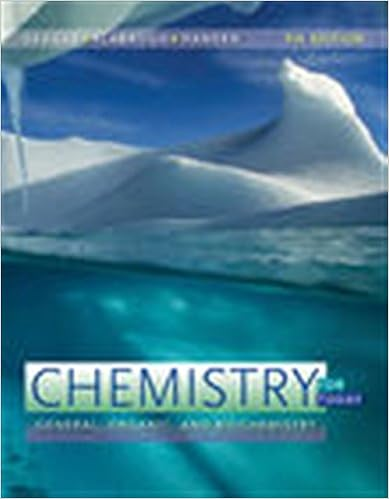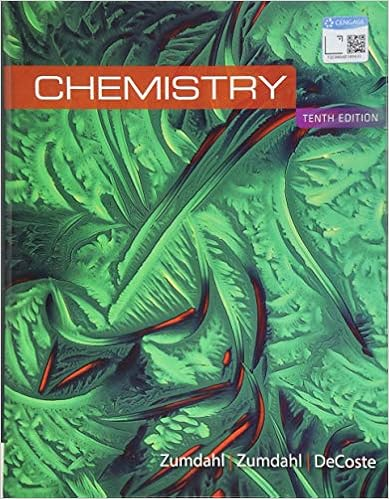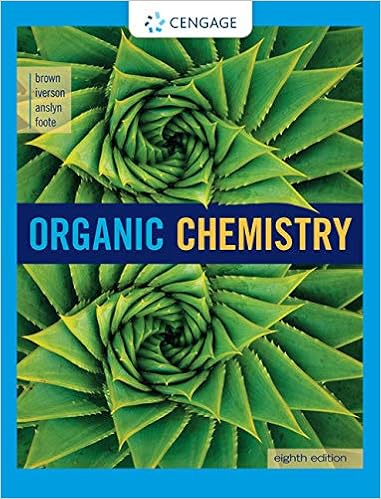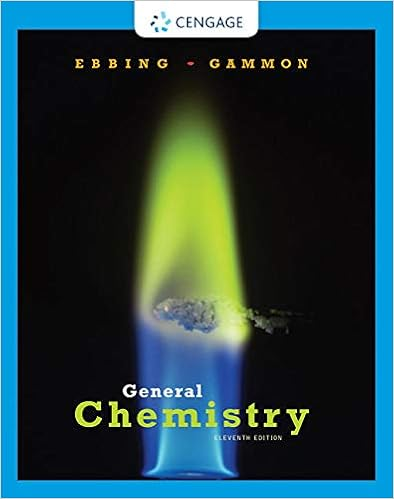# According to raoults law which statement is false a

• Notes
• 14
• 20% (10) 2 out of 10 people found this document helpful

Course Hero uses AI to attempt to automatically extract content from documents to surface to you and others so you can study better, e.g., in search results, to enrich docs, and more. This preview shows page 7 - 11 out of 14 pages.

32.According to Raoult's law, which statement isfalse?A. The vapor pressure of a solvent over a solution decreases as its mole fraction increases.B. The solubility of a gas increases as the temperature decreases.C. The vapor pressure of a solvent over a solution is less than that of pure solvent.D. The greater the pressure of a gas over a solution the greater its solubility.E.Ionic solutes dissociate in solution causing an enhancement of all colligative properties.
33.Which of the following aqueous solutions has the highest boiling point?
7
##### We have textbook solutions for you!The document you are viewing contains questions related to this textbook.
Chapter 7 / Exercise 7.80
Chemistry for Today: General, Organic, and Biochemistry
Seager/SlabaughExpert VerifiedBrowse all Textbook Solutions
34.Calculate the percent by mass of potassium nitrate in a solution made from 45.0 g KNO3and 295 mL ofwater. The density of water is 0.997 g/mL.
35.Calculate the molality of a 20.0% by mass ammonium sulfate (NH4)2SO4solution. The density of thesolution is 1.117 g/mL.
36.Arrange the following aqueous solutions in order of increasing boiling points.0.050 m Mg(NO3)2; 0.100 m ethanol; 0.090 m NaClA. Mg(NO3)2< NaCl < ethanolB. ethanol < Mg(NO3)2< NaClC. ethanol < NaCl < Mg(NO3)2D. NaCl < ethanol < Mg(NO3)2E. Mg(NO3)2< ethanol < NaCl37.The density of a 20.3 M CH3OH (methanol) solution is 0.858 g/mL. What is the molality of this solution?H2O is the solvent.A. 17.4 mB. 20.8 mC. 23.7 mD. 70.0 mE.97.6 m
38.Calculate the approximate freezing point of a solution made from 21.0 g NaCl and 1.00×102g of H2O.[Kfof water is 1.86°C/m.]
8
39.What is the molarity of a solution of 10% by mass cadmium sulfate, CdSO4(molar mass = 208.46 g/mol)by mass? The density of the solution is 1.10 g/mL.
40.The osmotic pressure of a 0.010 M MgSO4solution at 25°C is 0.318 atm. Calculatei, the van't Hofffactor, for this MgSO4solution.
41.The solubility of gases in water usually decreases withA. increasing pressure.B. increasing temperature.C.decreasing temperature.
42.Which of the following compounds should be soluble in CCl4?
43.What is the percent CdSO4by mass in a 1.0 molal aqueous CdSO4solution?
44.Calculate the molality of 6.0 M H2SO4solution. The density of the solution is 1.34 g/mL.
9
45.Calculate the molality of a solution containing 14.3 g of NaCl in 42.2 g of water.A.2.45×10-4B.5.80×10-4C.2.45×10-1D. 103 mE. 5.80 mmmm
46.What volume of water should be added to 600. mL of ethanol in order to have a solution that boils at95.0°C?[For ethanol, Kb= 1.22°C/m, density = 0.789 g/cm3, boiling point = 78.4°C]

Course Hero member to access this document

Course Hero member to access this document

End of preview. Want to read all 14 pages?

Course Hero member to access this document

Term
Fall
Professor
STAFF
Tags
Solvent, Vapor pressure
##### We have textbook solutions for you!
The document you are viewing contains questions related to this textbook.The document you are viewing contains questions related to this textbook.
Chapter 7 / Exercise 7.80
Chemistry for Today: General, Organic, and Biochemistry
Seager/SlabaughExpert Verified
•••